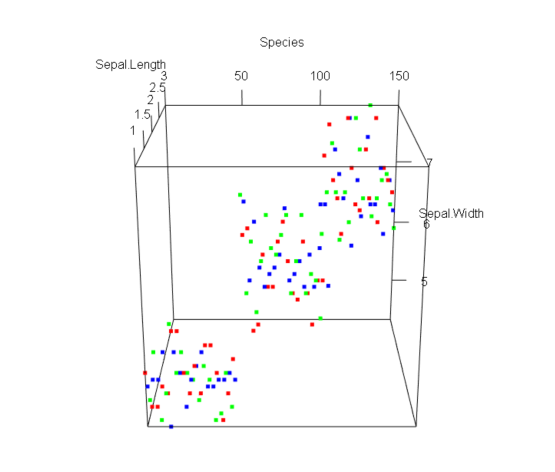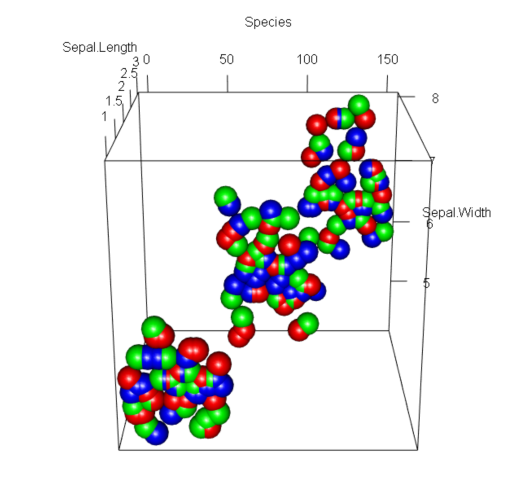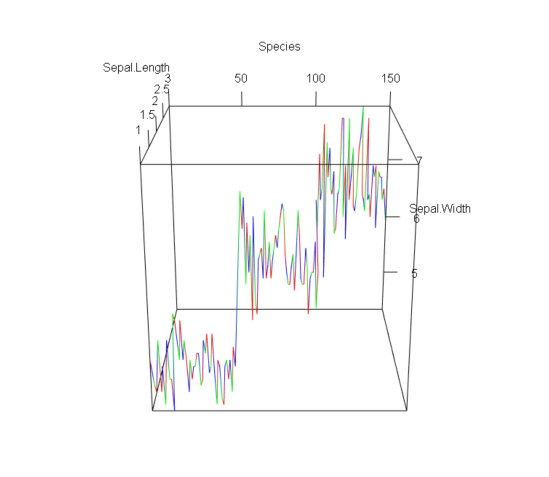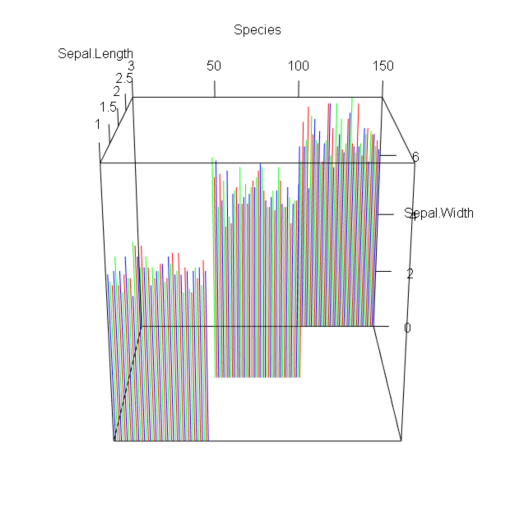Open in App
Not now

# How to create 3D plot for iris flower dataset with rgl package in R?

• Last Updated : 26 Dec, 2022

In R programming, the rgl() package is used to visualize the datasets in 3-Dimensions. It contains many 3D plotting functions, among them most widely functions used to plot 3d graphs include plot3d(), bgplot3d(), open3d(), planes3d(), points3d() etc. Each package mentioned here have specific usage but to scatter plot the given dataset we use plot3d() function in rgl package.

Iris Dataset is a built-in dataset in R which enables the R programmers to work on it without explicitly loading it into the R workspace and it contains five columns/fields namely Sepal.Length, Sepal.Width, Petal.Length, Petal.Width and Species.

Syntax : plot3d(x, y, z, xlab, ylab, zlab, type, col, add, size, radius, aspect,….)

• x, y, z – the vectors of points to be plotted on the x-axis, y-axis, and z-axis respectively.
• xlab, ylab, zlab – the names/labels which are used to display alongside coordinates in the plotted graph.
• type – used to define the type of scatter points we want to visualize like ‘s’ – for spheres, ‘l’ – for line, ‘p’ – for points(default), ‘h’ – for line segments
• col – used to define the color for each vector or for the whole graph(default=black)
• add  – used to restrict the permission of adding points to an existing plot(default=True)
• size – used to set size for plotted points
• aspect – used to adjust the aspect ratio of the graph.

To start with the code, we need to install and load rgl library. For that run the below command.

## R

 `# install required package` `install.packages``(``'rgl'``)` `# load that install package` `library``(rgl)`

Once we load the library, Now we can load the in-built iris dataset as well.

## R

 `# load iris dataset` `data``(iris)`

Now we can start the 3D visualization using plot3D function.

### Using type ‘p’

In this plot, we will use type = p, which means the plot locate the dataset in the form of points.

## R

 `# plot 1 ` `rgl::``plot3d``(iris\$Species,iris\$Sepal.Length,iris\$iris.Width,` `            ``xlab=``"Species"``,ylab=``"Sepal.Length"``,zlab=``"Sepal.Width"``,` `            ``type=``'p'``,col=``c``(``'red'``,``'blue'``,``'green'``),aspect=``c``(1,1,1),size=5)`

Output:### Using type ‘s’

Now, let’s see the plot for the type – s, which means spheres. In this case we need to add a new parameter radius, to specify the radius of the spheres.

## R

 `# plot 2` `rgl::``plot3d``(iris\$Species,iris\$Sepal.Length,iris\$iris.Width,` `            ``xlab=``"Species"``,ylab=``"Sepal.Length"``,zlab=``"Sepal.Width"``,` `            ``type=``'s'``,col=``c``(``'red'``,``'blue'``,``'green'``),aspect=``c``(1,1,1),radius=4)`

Output:### Using type ‘l’

Let’s see the case of type = l, it means line. The plot will automatically joins the points in the form of line which are 3D in nature.

## R

 `# plot 3` `rgl::``plot3d``(iris\$Species,iris\$Sepal.Length,iris\$iris.Width,` `            ``xlab=``"Species"``,ylab=``"Sepal.Length"``,zlab=``"Sepal.Width"``,` `            ``type=``'l'``,col=``c``(``'red'``,``'blue'``,``'green'``),aspect=``c``(1,1,1))`

Output:### Using type ‘h’

Let’s see the case of type = h, it means line segment. The plot will form line segment in 3D nature.

## R

 `# plot 4` `rgl::``plot3d``(iris\$Species,iris\$Sepal.Length,iris\$iris.Width,` `            ``xlab=``"Species"``,ylab=``"Sepal.Length"``,zlab=``"Sepal.Width"``,` `            ``type=``'h'``,col=``c``(``'red'``,``'blue'``,``'green'``),aspect=``c``(1,1,1),radius=4)`

Output:My Personal Notes arrow_drop_up
Related Articles